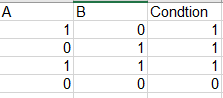# Challenge using If statement with both OR and AND conditionsHello,
Please I am trying to create this condition in XLS form such that:

1. If A2 is 1 and B2 is 0, my formula returns 1
2. If A2 is 0 and B2 is 1, my formula returns 1
3. If A2 is 1 and B2 is 1, my formula returns 1
4. If A2 is 0 and B2 is 0, my formula returns 0
The formula will look like this in excel =IF(OR(AND(A2=1,B2=1),A2=1,B2=1),1,0)
But in Kobo it is not working.
if(or(and(\${variable_name}=‘1’,\${variable_name}=‘2’),\${variable_name}=‘1’,\${variable_name}=‘7’),1,0)
Please if you can help me.

Hi @fya and welcome to the community!

Can you try this one:

`if (\${A}=1 or \${B}=1, 1, 0)`

Lets see if that works.

1 Like

Hi @hakan_cetinkaya
Thanks for your help, yes this works for either A or B but does not work when both A and B are selected because its multiple choice question.

Hi @fya,

Can you share the question in your mind with a simple example maybe, so community could understand it a little better?

1 Like

Hi @fya and @hakan_cetinkaya ,

I just built on your solution one step further - if this is in one question (as a multiple select) instead of two different questions, you can use this as a formula:

if (selected(\${Q1},‘a’) or selected(\${Q1},‘b’), 1, 0)

Let me know if that works!

Here’s a copy of the XLSForm to see it working:
A or B.xlsx (10.3 KB)

Hope that helps!
Janna

2 Likes

Hi @hakan_cetinkaya and @janna
Many thanks for your support.
The A or B excel is exactly what I am looking for.
Thanks again1 Like